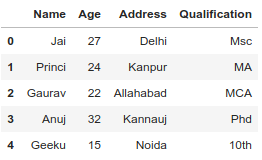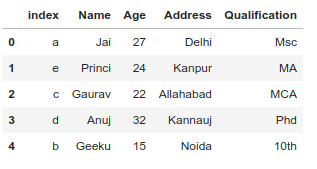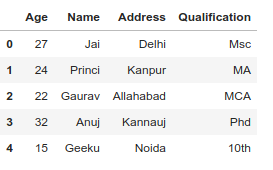# Reset Index in Pandas Dataframe

Let’s discuss how to reset index in Pandas DataFrame. Often We start with a huge dataframe in Pandas and after manipulating/filtering the dataframe, we end up with much smaller dataframe.

When we look at the smaller dataframe, it might still carry the row index of the original dataframe. If the original index are numbers, now we have indexes that are not continuous. Well, pandas has `reset_index()` function. So to reset the index to the default integer index beginning at 0, We can simply use the `reset_index()` function.

So let’s see the different ways we can reset the index of a DataFrame.

First see original DataFrame.

 `# Import pandas package ` `import` `pandas as pd ` `   `  `# Define a dictionary containing employee data ` `data ``=` `{``'Name'``:[``'Jai'``, ``'Princi'``, ``'Gaurav'``, ``'Anuj'``, ``'Geeku'``], ` `        ``'Age'``:[``27``, ``24``, ``22``, ``32``, ``15``], ` `        ``'Address'``:[``'Delhi'``, ``'Kanpur'``, ``'Allahabad'``, ``'Kannauj'``, ``'Noida'``], ` `        ``'Qualification'``:[``'Msc'``, ``'MA'``, ``'MCA'``, ``'Phd'``, ``'10th'``] } ` ` `  `# Convert the dictionary into DataFrame  ` `df ``=` `pd.DataFrame(data) ` ` `  `df `

Output:Example #1: Make Own Index Without Removing Default index.

 `# Import pandas package ` `import` `pandas as pd ` `   `  `# Define a dictionary containing employee data ` `data ``=` `{``'Name'``:[``'Jai'``, ``'Princi'``, ``'Gaurav'``, ``'Anuj'``, ``'Geeku'``], ` `        ``'Age'``:[``27``, ``24``, ``22``, ``32``, ``15``], ` `        ``'Address'``:[``'Delhi'``, ``'Kanpur'``, ``'Allahabad'``, ``'Kannauj'``, ``'Noida'``], ` `        ``'Qualification'``:[``'Msc'``, ``'MA'``, ``'MCA'``, ``'Phd'``, ``'10th'``] } ` ` `  `index ``=` `{``'a'``, ``'b'``, ``'c'``, ``'d'``, ``'e'``} ` ` `  `# Convert the dictionary into DataFrame  ` `df ``=` `pd.DataFrame(data, index) ` ` `  `# Make Own Index as index ` `# In this case default index is exist  ` `df.reset_index(inplace ``=` `True``) ` ` `  `df `

Output:Example #2: Make Own Index and Removing Default index.

 `# Import pandas package ` `import` `pandas as pd ` `   `  `# Define a dictionary containing employee data ` `data ``=` `{``'Name'``:[``'Jai'``, ``'Princi'``, ``'Gaurav'``, ``'Anuj'``, ``'Geeku'``], ` `        ``'Age'``:[``27``, ``24``, ``22``, ``32``, ``15``], ` `        ``'Address'``:[``'Delhi'``, ``'Kanpur'``, ``'Allahabad'``, ``'Kannauj'``, ``'Noida'``], ` `        ``'Qualification'``:[``'Msc'``, ``'MA'``, ``'MCA'``, ``'Phd'``, ``'10th'``] } ` ` `  `# Create own index ` `index ``=` `{``'a'``, ``'b'``, ``'c'``, ``'d'``, ``'e'``} ` ` `  `# Convert the dictionary into DataFrame  ` `# Make Own Index and Removing Default index ` `df ``=` `pd.DataFrame(data, index) ` ` `  `df `

Output:Example 3: Reset own index and make default index as index.

 `# Import pandas package ` `import` `pandas as pd ` `   `  `# Define a dictionary containing employee data ` `data ``=` `{``'Name'``:[``'Jai'``, ``'Princi'``, ``'Gaurav'``, ``'Anuj'``, ``'Geeku'``], ` `        ``'Age'``:[``27``, ``24``, ``22``, ``32``, ``15``], ` `        ``'Address'``:[``'Delhi'``, ``'Kanpur'``, ``'Allahabad'``, ``'Kannauj'``, ``'Noida'``], ` `        ``'Qualification'``:[``'Msc'``, ``'MA'``, ``'MCA'``, ``'Phd'``, ``'10th'``] } ` ` `  `# Create own index ` `index ``=` `{``'a'``, ``'b'``, ``'c'``, ``'d'``, ``'e'``} ` ` `  `# Convert the dictionary into DataFrame  ` `df ``=` `pd.DataFrame(data, index) ` ` `  `# remove own index with default index ` `df.reset_index(inplace ``=` `True``, drop ``=` `True``) ` ` `  `df `

Output:Example #4: Make a column of dataframe as index with remove default index.

 `# Import pandas package ` `import` `pandas as pd ` `   `  `# Define a dictionary containing employee data ` `data ``=` `{``'Name'``:[``'Jai'``, ``'Princi'``, ``'Gaurav'``, ``'Anuj'``, ``'Geeku'``], ` `        ``'Age'``:[``27``, ``24``, ``22``, ``32``, ``15``], ` `        ``'Address'``:[``'Delhi'``, ``'Kanpur'``, ``'Allahabad'``, ``'Kannauj'``, ``'Noida'``], ` `        ``'Qualification'``:[``'Msc'``, ``'MA'``, ``'MCA'``, ``'Phd'``, ``'10th'``] } ` ` `  `# Create own index ` `index ``=` `{``'a'``, ``'b'``, ``'c'``, ``'d'``, ``'e'``} ` ` `  `# Convert the dictionary into DataFrame  ` `df ``=` `pd.DataFrame(data, index) ` ` `  `# set index any column of our DF and ` `# remove default index ` `df.set_index([``'Age'``], inplace ``=` `True``) ` ` `  `df `

Output:Example 5: Make a column of dataframe as index without remove default index.

 `# Import pandas package ` `import` `pandas as pd ` `   `  `# Define a dictionary containing employee data ` `data ``=` `{``'Name'``:[``'Jai'``, ``'Princi'``, ``'Gaurav'``, ``'Anuj'``, ``'Geeku'``], ` `        ``'Age'``:[``27``, ``24``, ``22``, ``32``, ``15``], ` `        ``'Address'``:[``'Delhi'``, ``'Kanpur'``, ``'Allahabad'``, ``'Kannauj'``, ``'Noida'``], ` `        ``'Qualification'``:[``'Msc'``, ``'MA'``, ``'MCA'``, ``'Phd'``, ``'10th'``] } ` ` `  `# Create own index ` `index ``=` `{``'a'``, ``'b'``, ``'c'``, ``'d'``, ``'e'``} ` ` `  `# Convert the dictionary into DataFrame  ` `df ``=` `pd.DataFrame(data, index) ` ` `  `# set any column as index ` `# Here we set age column as index ` `df.set_index([``'Age'``], inplace ``=` `True``) ` ` `  `# reset index without removing default index ` `df.reset_index(level ``=``[``'Age'``], inplace ``=` `True``) ` ` `  `df `

Output:My Personal Notes arrow_drop_upCheck out this Author's contributed articles.

If you like GeeksforGeeks and would like to contribute, you can also write an article using contribute.geeksforgeeks.org or mail your article to contribute@geeksforgeeks.org. See your article appearing on the GeeksforGeeks main page and help other Geeks.

Please Improve this article if you find anything incorrect by clicking on the "Improve Article" button below.

Article Tags :

Be the First to upvote.

Please write to us at contribute@geeksforgeeks.org to report any issue with the above content.